# Pre University Courses

Solutions and Acids and Bases Selected sections of the textbook.

This concept organizer is an important resources. Please print off this page and look up the diagrams in your textbook for ion-dipole attraction.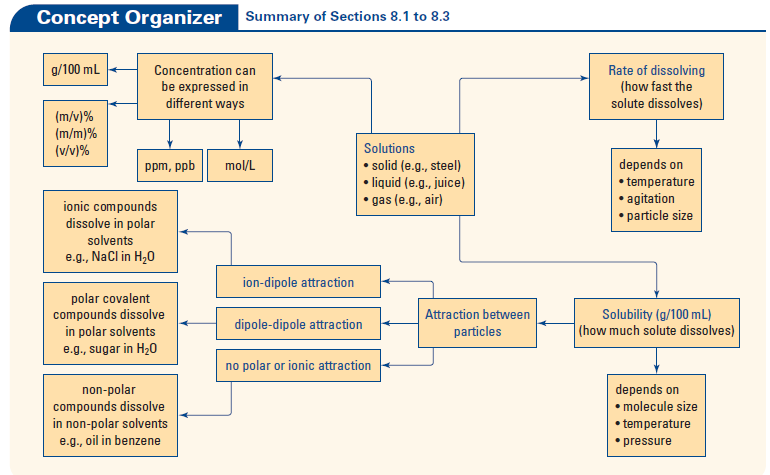pH Value of everyday objects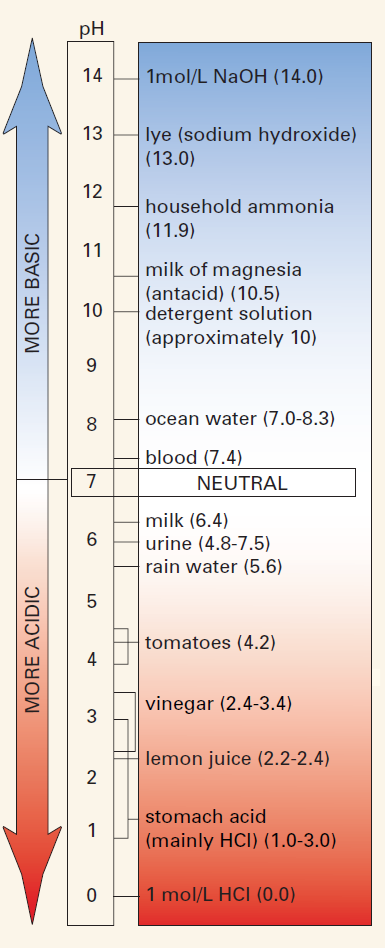pH Calculations

### All aqueous solutions contain ions ( which is a positively or negatively charged particle that results from a neutral atom or group of atoms giving up or gaining electrons).  In this case are are investigating when both strong and weak bases are mixed with water and determining the pH value. Which means the "p" stands for Power of the hydronium (or hydrogen) ion.

Dissociation of of an acid means when acid is mixed with water ( H2 O ) a specific formation of ions occurs.   For example when hydrochloric acid is mixed with water, the following reaction occurs.   The concentration of

HCl + H2 O --->  H ­3 O +   +    Cl -

When you measure the dissociation you are given an exponential notation value of the hydronium ions in moles per liter.

To get the pH value you take your moles per liter value and take the - log value to get the log value which is also the pH value. Many of you may have already of this value before in your everyday lives.

Calculating the pH value.

pH  =   -log [H­3O + ]

Notice from the table below, the strong acid has a very low pH value, and strong base has a value of 14. This means the pH range is between 0 and 14.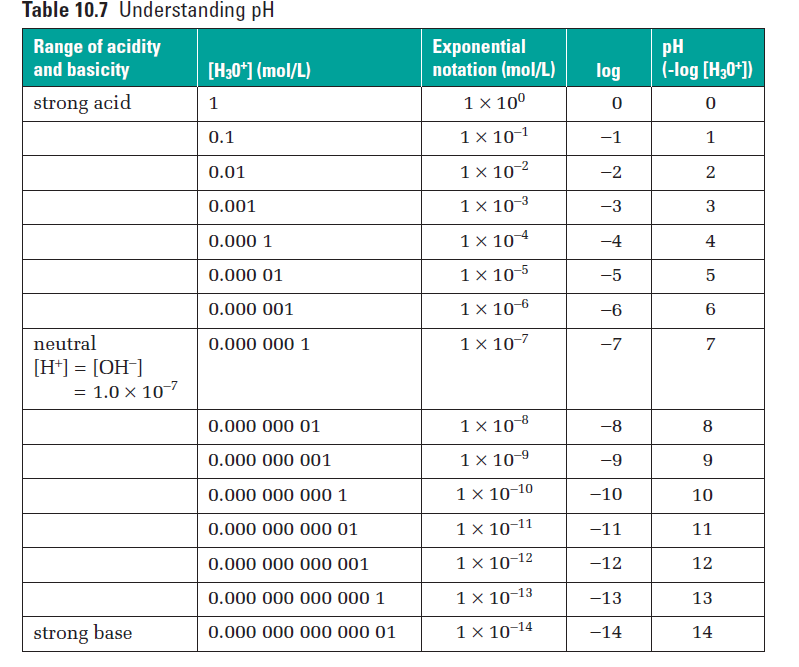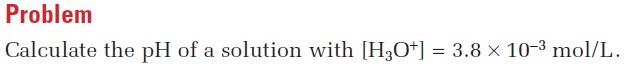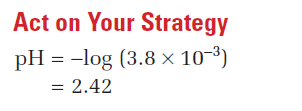Practice Problems from text p. 389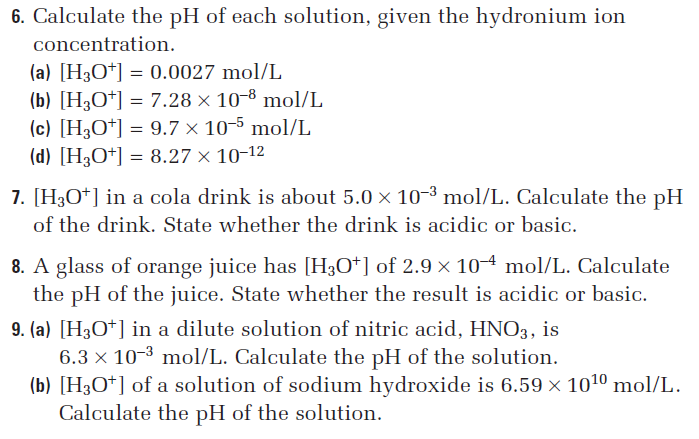Theory of Acid and Bases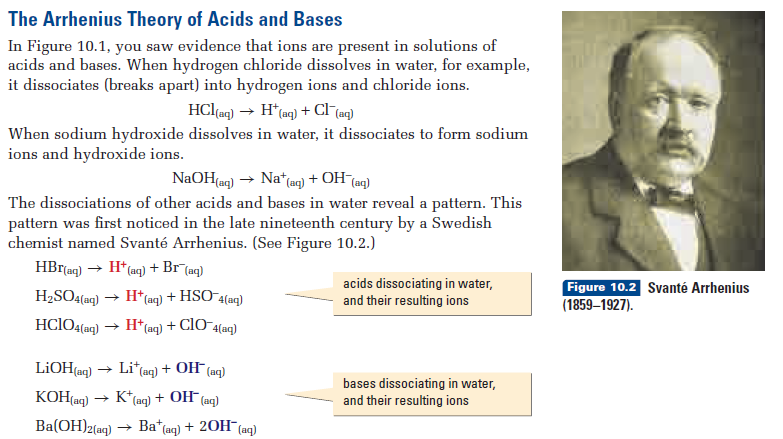Review for the EXAM- Name the acids above- and write them in your Revision book